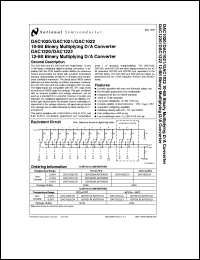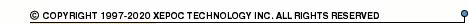More than478 737 registered clientsDAC1020 series datasheets. Manufacturer: National Semiconductor.

 DAC1020LCJ 10-Bit Binary Multiplying D/A Converter Datasheet*) DAC1020LCN 10-Bit Binary Multiplying D/A Converter Datasheet*) DAC1020LCV 10-Bit Binary Multiplying D/A Converter Datasheet*) DAC1020LCVX 10-Bit Binary Multiplying D/A Converter Datasheet*) DAC1020LJ 10-Bit Binary Multiplying D/A Converter Datasheet*) DAC1020LCN 10-bit binary multiplying D/A converter in 16-pin DIP package. Operational temperature range from 0°C to 70°C. Datasheet*) DAC1020LCV 10-bit binary multiplying D/A converter in 20-pin PLCC package. Operational temperature range from 0°C to 70°C. Datasheet*) DAC1020LIV 10-bit binary multiplying D/A converter in 20-pin PLCC package. Operational temperature range from -40°C to 85°C. Datasheet*) DAC1021LCN 10-bit binary multiplying D/A converter in 16-pin DIP package. Operational temperature range from 0°C to 70°C. Datasheet*) DAC1022LCN 10-bit binary multiplying D/A converter in 16-pin DIP package. Operational temperature range from 0°C to 70°C. Datasheet*) DAC1220LCJ 12-bit binary multiplying D/A converter in 18-pin DIP package. Operational temperature range from -40°C to 85°C. Datasheet*) DAC1220LCN 12-bit binary multiplying D/A converter in 18-pin DIP package. Operational temperature range from 0°C to 70°C. Datasheet*) DAC1222LCJ 12-bit binary multiplying D/A converter in 18-pin DIP package. Operational temperature range from -40°C to 85°C. Datasheet*) DAC1222LCN 12-bit binary multiplying D/A converter in 18-pin DIP package. Operational temperature range from 0°C to 70°C. Datasheet*) DAC1020 10-Bit, 12-Bit Binary Multiplying D/A Converter Datasheet*) DAC1021 10-Bit, 12-Bit Binary Multiplying D/A Converter Datasheet*) DAC1022 10-Bit, 12-Bit Binary Multiplying D/A Converter Datasheet*) DAC1222 10-Bit, 12-Bit Binary Multiplying D/A Converter Datasheet*)